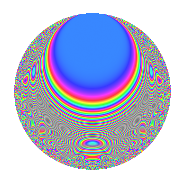# Properties

 Label 349.2.eLevel 349 Weight 2 Character orbit e Rep. character $$\chi_{349}(123,\cdot)$$ Character field $$\Q(\zeta_{6})$$ Dimension 58 Newform subspaces 1 Sturm bound 58 Trace bound 0

# Related objects

## Defining parameters

 Level: $$N$$ = $$349$$ Weight: $$k$$ = $$2$$ Character orbit: $$[\chi]$$ = 349.e (of order $$6$$ and degree $$2$$) Character conductor: $$\operatorname{cond}(\chi)$$ = $$349$$ Character field: $$\Q(\zeta_{6})$$ Newform subspaces: $$1$$ Sturm bound: $$58$$ Trace bound: $$0$$

## Dimensions

The following table gives the dimensions of various subspaces of $$M_{2}(349, [\chi])$$.

Total New Old
Modular forms 62 62 0
Cusp forms 58 58 0
Eisenstein series 4 4 0

## Trace form

 $$58q - 3q^{2} + 27q^{4} + 2q^{5} - 29q^{9} + O(q^{10})$$ $$58q - 3q^{2} + 27q^{4} + 2q^{5} - 29q^{9} - q^{12} - 3q^{13} - 10q^{14} + q^{15} - 29q^{16} - 10q^{17} + 15q^{18} - 9q^{19} - 3q^{22} - 17q^{23} - 48q^{24} - 29q^{25} - 4q^{26} + 18q^{27} - 2q^{29} + 9q^{30} + 32q^{31} + 9q^{32} - 12q^{33} - 63q^{34} + 24q^{36} - 16q^{37} + 54q^{40} - 10q^{41} - 15q^{42} - 45q^{43} + 18q^{44} - 2q^{45} + 27q^{46} + 6q^{48} + 35q^{49} + 6q^{50} - 14q^{51} + 27q^{54} + 24q^{55} + 11q^{56} - 29q^{57} - 18q^{59} + 116q^{60} - 9q^{62} - 21q^{63} - 132q^{64} + 130q^{66} + 58q^{67} + 42q^{69} + 40q^{70} - 24q^{71} + 72q^{72} - 6q^{73} + 30q^{74} - 58q^{75} + 37q^{76} - 4q^{77} - 33q^{78} - 40q^{80} - 81q^{81} + 21q^{82} + 12q^{83} + 18q^{84} - 11q^{85} - 126q^{86} - 42q^{87} - 50q^{88} + 3q^{89} - 12q^{90} - 28q^{91} - 120q^{92} + 31q^{93} + 29q^{94} + 60q^{95} - 120q^{96} - 15q^{97} + 39q^{99} + O(q^{100})$$

## Decomposition of $$S_{2}^{\mathrm{new}}(349, [\chi])$$ into newform subspaces

Label Dim. $$A$$ Field CM Traces $q$-expansion
$$a_2$$ $$a_3$$ $$a_5$$ $$a_7$$
349.2.e.a $$58$$ $$2.787$$ None $$-3$$ $$0$$ $$2$$ $$0$$

## Hecke Characteristic Polynomials

There are no characteristic polynomials of Hecke operators in the database